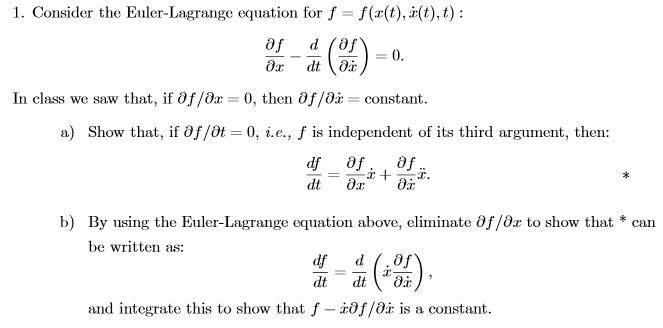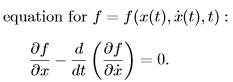# Euler-Lagrange equation application

• Ascendant78

## Homework Statement## Homework Equations## The Attempt at a Solution

I have tried manipulating the equation a few different ways, but the Euler-Lagrange and the one I'm supposed to show for a) is so different that I just can't seem to work. Can someone please point me in the right direction here?

I think you don't need the Euler-Lagrange equation for (a). That looks like the standard derivative, just calculate it.

Well, just calculating the derivative, I'm not sure why it is coming out the way it is? I get that the "t" component would be zeros, cancelling out its parts. However, I'm not sure what derivative/partial derivative rules are being applied to get the equation to look like the one asked for in a)?

Think chain rule. If you still can't figure it out, show us your attempt.